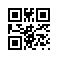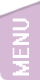[精讚] [會員登入]
30

### Python 特色寫法整理(1)：給有程式基礎的人

python初心者：Python 特色寫法整理

#### 分享此文連結//n.sfs.tw/15841

##### 2022-05-18 22:39:42 By 張○○### 自動目錄

python和其它的直譯式語言來說，(如php、perl)大同小異，雖然我很抗拒，但還是稍微摸了一下。

### 結構

1. 不用大括號，採用縮排來分段。縮排可以用空白或是tab來決定，官方建議用 4 個空格縮排，不要用 tab 鍵。 一個縮排段落內的縮排方式與數量必須維持一致。 例如你決定用四個空白當縮排，那整個文檔的縮排就只能用四個空白，三個空白或五個空白都會報錯。我記得以前學過 true basic 也是這個架構。

2. 運算符(+-*/)、'='的前後請加上空白，雖然不會報錯，但會出現警告。

3. 行尾不必有分號 ';' ，加了也無所謂只是會警告，行前後不要有多餘的空白，否則會出錯或警告

4.  使用 # 作為註解，等於c-like語言的  '//' ；用三個雙引號 """ ... """ 等於c-like語言的  '/* */' 以大範圍註解。

5. 變數前不用加錢字號 '\$'

6. python 算是強型別，有int, float, string, decimal, complex 等等，你無法忽略型別，例如你要印數字，你得把他轉成字串。

7. python 具有布林值 True/ False，首字必須大寫才是布林值。例如 a = True

8. 函數可回傳多值、多型態，不像一般語言只能回傳單值。

9. 可快速指定變數，例如 a, b, c = 1, 2, 3

### 運算子及資料結構

#### 值得注意的運算子

x * y  乘法，字串的乘法就是複製

print('x'*10)  #xxxxxxxxxx
x // y  整數除法

x % y 餘數除法
x ** y  次方

#### 字串拆解

s = 'Onetwothree'
s1= s[1:] #netwothree
s2= s[3:-1] #twothre
s3= s.upper() + s[1:] #Onetwothree 首字大寫

#### 串列 List

squares = [1, 4, 9, 16, 25]
squares #1
squares[-1] #25
squares[-3:] # 新list [9, 16, 25]
squares + [36, 49, 64, 81] #接合  [1, 4, 9, 16, 25, 36, 49, 64, 81]
squares.insert(2, 22) # 在第2個位置加插入數字22，原本序號2後的數值往後移一位
squares = 36 # 指定值
squares.append(216) # 加一個值在最後面
squares[:] = [] # 清空串列
clear(squraes) # 清空串列
squares[2:4] = [] # 清空第2,3 兩個位置的值，注意第4個位置的值沒有清掉。
del(squares) # 清空第2兩個位置的值
len(squares) # 串列長度
squares.reverse()  # 前後顛倒
print(squares)  # 印出串列

#### 唯讀串列 Tuple

solar = ('earth','jupiter', ['io', 'ganymede', 'europa'])

print(solar)  #earth
print(solar)  #europa
solar='callisto'
print(solar)  #callisto <== 唯讀串列中的串列是可以修改的
solar='mars'  #報錯，唯讀串列不能修改

squares = [1, 4, 9, 16, 25]
sqr= tuple(squares)

#### 字典 dict

solar = {'earth': 'moon', 'jupiter': ['io', 'ganymede', 'europa'], 'pluto': 'charon', 'sun': 8}
solar['earth'] # moon
solar['jupiter'] # ganymede
len(solar) # 4 長度
del(solar['pluto']) #刪除一個項目
solar['mars']=['deimos', 'phobos']  #加入一個項目
print(solar)   # 印出集合

#### 集合 set

a = set([1,3,6,7,3])
b = set([1,2,5,6])
print(a)    #{1, 3, 6, 7} <== 移除重覆的3
print(a&b)  #{1, 6}
print(a|b)  #{1, 2, 3, 5, 6, 7}
print(a-b)  #{3, 7}
print(b-a)  #{2, 5}
print(a^b)  #{2, 3, 5, 7}

### 流程控制

#### if... elif ... else

if 2 > 5:
print('2 > 5')
elif 2 < 5:
print('2 < 5')
else:
print('2 == 5')

#### match ... case

x = input("輸入數字: ")
match x:
case 1: print('星期一')  #只有一行指令可寫同一行
case 2:
print('星期二')  # 不寫同一行要縮排
case 3: pass  # 啥都不做
case '4': print('星期四')
case 5.0: print('星期五')
case 6 | 7: print('Weekend')
case _: print("輸入錯誤")  # 其它項目用 _

<<啥都沒發生>>

#### for ... in

solar = {'earth': 'moon', 'jupiter': ['io', 'ganymede', 'europa'], 'pluto': 'charon', 'sun': 8}
for it in solar:
print(it + ': ', solar[it])

earth:  moon
jupiter:  ['io', 'ganymede', 'europa']
pluto:  charon
sun:  8

x = "字串a1;"  # list(x)=['字', '串', 'a', '1', ';']
for i in range(len(x)):
print(x[i])

a
1
;

#### range() 函數來取代 for(;;)

range是一個類別，可以產生連續的「串列類別物件」，注意，不是產生「串列」

z = [0, 1, 2, 3, 4]  #串列
print(z) #[0, 1, 2, 3, 4] <== 印出串列
x = range(5)  #range物件
print(x, type(x))  #range(0, 5) <class 'range'>  <==印出類別本身
print(list(x))  #[0, 1, 2, 3, 4]

x = range(5)  #[0, 1, 2, 3, 4]  <== 注意不包括5
x = range(1, 5)  #[1, 2, 3, 4]  <== 注意不包括5
x = range(0, 6, 2)  #[0, 2, 4]  <== 注意不包括6
x = range(0, 10, 3)  #[0, 3, 6, 9]
x = range(0, -5, -1)  #[0, -1, -2, -3, -4]
x = range(0) #[]
x = range(1, 0)  #[]
x = range(1, 0, -1)  #

python沒有c-like的for(;;) 寫法，用range來取代。

#### 使用range來快速產生串列

a1 = [i for i in range(5)]  #[0, 1, 2, 3, 4] <== 注意不包括5

a2 = [i for i in range(0,10,2)]  #[0, 2, 4, 6, 8] 產生偶數

a3 = [i for i in range(1,10,2)]  #[1, 3, 5, 7, 9] 產生奇數

a4 = [i for i in a3 if i > 3]  #[5, 7, 9] 限制條件

planet = ['mecury','venus','earth','mars','jupiter']
# 首字大寫
a5 = [i.title() for i in planet ]  #['Mecury', 'Venus', 'Earth', 'Mars', 'Jupiter']

# 串列中有字元'e'的前面加上 'the '
a6 = ['the ' + i for i in planet if 'e' in i]  #['the mecury', 'the venus', 'the earth', 'the jupiter']

#### raise, try...except

python中沒有 try... catch...finally，但有差不多的 try...except錯誤嘗試控制

n = 10
m = 0
try:
print(n/m)
except:
print('除以0錯誤')

### 結論

python的指令和功能不只這些，只是我做了簡要的整理，對於有其它程式基礎的人，希望這篇會對你有幫助。

END

### 你可能感興趣的文章

Python 特色寫法整理(1)：給有程式基礎的人 python初心者：Python 特色寫法整理

[Python3+Line] 使用 line notify 傳送訊息、圖片和貼圖 python+ line notify 傳送訊息、圖片和貼圖

Python 特色寫法整理(2)：給有程式基礎的人 python初心者：Python 特色寫法整理，函數、類別、引用等等

>>

[無留言]

### 隨機好文

HP SAS硬碟leds燈號說明 HP SAS硬碟leds燈號(hp g7/g6系統適用)說明

APACHE的記錄檔格式 LogFormat 語法 在APACHE中有定義一些記錄的語法模版 在 /etc/httpd/conf/httpd.conf 中： LogForm

[AS3] 變數型態 基本類型宣告 as3 有下列幾種基本類型：string, int, number, object, boolean, n